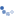# Temperature deformation in construction stage

Question:

Hi all,

It is a simple cantilever beam subjected to a temperature load (stage 1, -176°C) and a compression axial force (stage 2, 1000 kN).

At the end of the stages we expect this axial displacement:

Stage 1) DL1 = alfa x DT x L = 5.45E-6 [1/°C] x 176 [°C] x 1000 [mm] = 0.9592 mm

Stage 2) DL2 = DL1 + N/EA x (L - DL1) =0.9592 mm + 1000000 [N] / (200000 [N/m2] x 100 [mm2]) x (1000 [mm] - 0.9592 [mm]) = 0.9592 mm + 49.952 mm = 50.91124 mm

Note that we considered the new length of the beam for the calculation of the dispalcement due to the force. The length is different beacuse of the temperature load in the previous stage.

Midas civil gives an another value: 50.9592 mm.
We noted that it doesn't update the new length of the beam:
Stage 1) DL1 = 0.9592 mm
Stage 2) DL2 = DL1+ N/EA x L = 0.9592mm + 50mm = 50.9592mm

So it seems that the construction stage analysis is a simple linear combination of effect and not consider the updates of the geometry of structures.

Can you check it or explain us why?
Thank you very much

Hello,

Thank you for sending your question.
In midas Gen, linear analysis of beam element is based on the assumption of the small strain-small rotation. Therefore, the strain caused by temperature load of step1 is ignored in the deformation calculation of the step2.
Thank you.

Best regards,
Jihong Hwang

Even setting the analysis as non linear, the results don't change:Why Midas does not take into account axial large displacement?

Thank you
regardsHello,